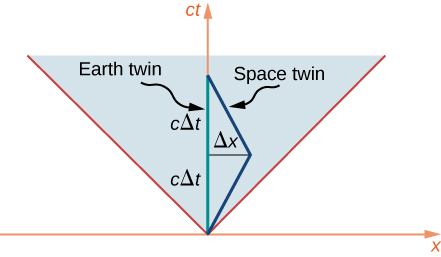# 5.5 The lorentz transformation  (Page 6/12)

 Page 6 / 12

For any event that has a space-like separation from the event at the origin, it is possible to choose a time axis that will make the two events occur at the same time, so that the two events are simultaneous in some frame of reference. Therefore, which of the events with space-like separation comes before the other in time also depends on the frame of reference of the observer. Since space-like separations can be traversed only by exceeding the speed of light; this violation of which event can cause the other provides another argument for why particles cannot travel faster than the speed of light, as well as potential material for science fiction about time travel. Similarly for any event with time-like separation from the event at the origin, a frame of reference can be found that will make the events occur at the same location. Because the relations

$\text{Δ}{s}_{AC}^{2}={\left({x}_{A}-{x}_{B}\right)}^{2}+{\left({x}_{A}-{x}_{B}\right)}^{2}+{\left({x}_{A}-{x}_{B}\right)}^{2}-{\left(c\text{Δ}t\right)}^{2}>0$

and

$\text{Δ}{s}_{AB}^{2}={\left({x}_{A}-{x}_{B}\right)}^{2}+{\left({x}_{A}-{x}_{B}\right)}^{2}+{\left({x}_{A}-{x}_{B}\right)}^{2}-{\left(c\text{Δ}t\right)}^{2}<0.$

are Lorentz invariant, whether two events are time-like and can be made to occur at the same place or space-like and can be made to occur at the same time is the same for all observers. All observers in different inertial frames of reference agree on whether two events have a time-like or space-like separation.

## The twin paradox seen in space-time

The twin paradox discussed earlier involves an astronaut twin traveling at near light speed to a distant star system, and returning to Earth. Because of time dilation, the space twin is predicted to age much less than the earthbound twin. This seems paradoxical because we might have expected at first glance for the relative motion to be symmetrical and naively thought it possible to also argue that the earthbound twin should age less.

To analyze this in terms of a space-time diagram, assume that the origin of the axes used is fixed in Earth. The world line of the earthbound twin is then along the time axis.

The world line of the astronaut twin, who travels to the distant star and then returns, must deviate from a straight line path in order to allow a return trip. As seen in [link] , the circumstances of the two twins are not at all symmetrical. Their paths in space-time are of manifestly different length. Specifically, the world line of the earthbound twin has length $2c\text{Δ}t,$ which then gives the proper time that elapses for the earthbound twin as $2\text{Δ}t.$ The distance to the distant star system is $\text{Δ}x=v\text{Δ}t.$ The proper time that elapses for the space twin is $2\text{Δ}\tau$ where

${c}^{2}\text{Δ}{\tau }^{2}=-\text{Δ}{s}^{2}={\left(c\text{Δ}t\right)}^{2}-{\left(\text{Δ}x\right)}^{2}.$

This is considerably shorter than the proper time for the earthbound twin by the ratio

$\begin{array}{cc}\hfill \frac{c\text{Δ}\tau }{c\text{Δ}t}& =\sqrt{\frac{{\left(c\text{Δ}t\right)}^{2}-{\left(\text{Δ}x\right)}^{2}}{{\left(c\text{Δ}t\right)}^{2}}}=\sqrt{\frac{{\left(c\text{Δ}t\right)}^{2}-{\left(v\text{Δ}t\right)}^{2}}{{\left(c\text{Δ}t\right)}^{2}}}\hfill \\ & =\sqrt{1-\frac{{v}^{2}}{{c}^{2}}}=\frac{1}{\gamma }.\hfill \end{array}$

consistent with the time dilation formula. The twin paradox is therefore seen to be no paradox at all. The situation of the two twins is not symmetrical in the space-time diagram. The only surprise is perhaps that the seemingly longer path on the space-time diagram corresponds to the smaller proper time interval, because of how $\text{Δ}\tau$ and $\text{Δ}s$ depend on $\text{Δ}x$ and $\text{Δ}t.$The space twin and the earthbound twin, in the twin paradox example, follow world lines of different length through space-time.

#### Questions & Answers

how does colour appear in thin films
in the wave equation y=Asin(kx-wt+¢) what does k and w stand for.
derivation of lateral shieft
Hi
Hi
hi
ALFRED
how are you?
hi
asif
hi
Imran
I'm fine
ALFRED
total binding energy of ionic crystal at equilibrium is
How does, ray of light coming form focus, behaves in concave mirror after refraction?
Refraction does not occur in concave mirror. If refraction occurs then I don't know about this.
Sushant
What is motion
Anything which changes itself with respect to time or surrounding
Sushant
good
Chemist
and what's time? is time everywhere same
Chemist
No
Sushant
how can u say that
Chemist
do u know about black hole
Chemist
Not so more
Sushant
DHEERAJ
These substance create harmful radiation like alpha particle radiation, beta particle radiation, gamma particle radiation
Sushant
But ask anything changes itself with respect to time or surrounding A Not any harmful radiation
DHEERAJ
explain cavendish experiment to determine the value of gravitational concept.
For the question about the scuba instructor's head above the pool, how did you arrive at this answer? What is the process?
as a free falling object increases speed what is happening to the acceleration
of course g is constant
Alwielland
acceleration also inc
Usman
which paper will be subjective and which one objective
jay
normal distributiin of errors report
Dennis
normal distribution of errors
Dennis
photo electrons doesn't emmit when electrons are free to move on surface of metal why?
What would be the minimum work function of a metal have to be for visible light(400-700)nm to ejected photoelectrons?
give any fix value to wave length
Rafi
40 cm into change mm
40cm=40.0×10^-2m =400.0×10^-3m =400mm. that cap(^) I have used above is to the power.
Prema
i.e. 10to the power -2 in the first line and 10 to the power -3 in the the second line.
Prema
there is mistake in my first msg correction is 40cm=40.0×10^-2m =400.0×10^-3m =400mm. sorry for the mistake friends.
Prema
40cm=40.0×10^-2m =400.0×10^-3m =400mm.
Prema
this msg is out of mistake. sorry friends​.
Prema
what is physics?
why we have physics
because is the study of mater and natural world
John
because physics is nature. it explains the laws of nature. some laws already discovered. some laws yet to be discovered.
Yoblaze
physics is the study of non living things if we added it with biology it becomes biophysics and bio is the study of living things tell me please what is this?
tahreem
physics is the study of matter,energy and their interactions
Buvanes
all living things are matter
Buvanes
why rolling friction is less than sliding friction
tahreem
thanks buvanas
tahreem
is this a physics forumByByBy